﻿ 舰船直流电机转速自稳定控制系统
 舰船科学技术2020, Vol. 42Issue (6): 137-140    DOI: 10.3404/j.issn.1672-7649.2020.06.027PDF

1. 重庆交通大学 交通运输学院，重庆 400074;
2. 重庆交通大学 航运与船舶工程学院，重庆 400074

Speed self-stabilization control system of ship DC motor
SHENG Jin-lu1,2, ZHANG Shou-bao2, WAN Li1, GUO Qiang2
1. School of Transportation, Chongqing Jiaotong University, Chongqing 400074, China;
2. School of Shipping and Ship Engineering, Chongqing Jiaotong University, Chongqing 400074, China
Abstract: A speed self-stabilization control system with strong self-control ability is proposed.A series of methods like reducing the control quantity of PID,introducing current cutoff negative feedback and optimizing the circuit are taking to improve the stability of the speed and to ensure the safety of the system during operation. The system can realize DC motor soft-start through soft control,over-current protection,anti-supply voltage fluctuation and other functions.The test proves that the system can make the motor run smoothly at a given speed.
Key words: self-stabilization     PID control     DC motor soft-start     over-current protection
0 引　言

1 系统硬件结构设计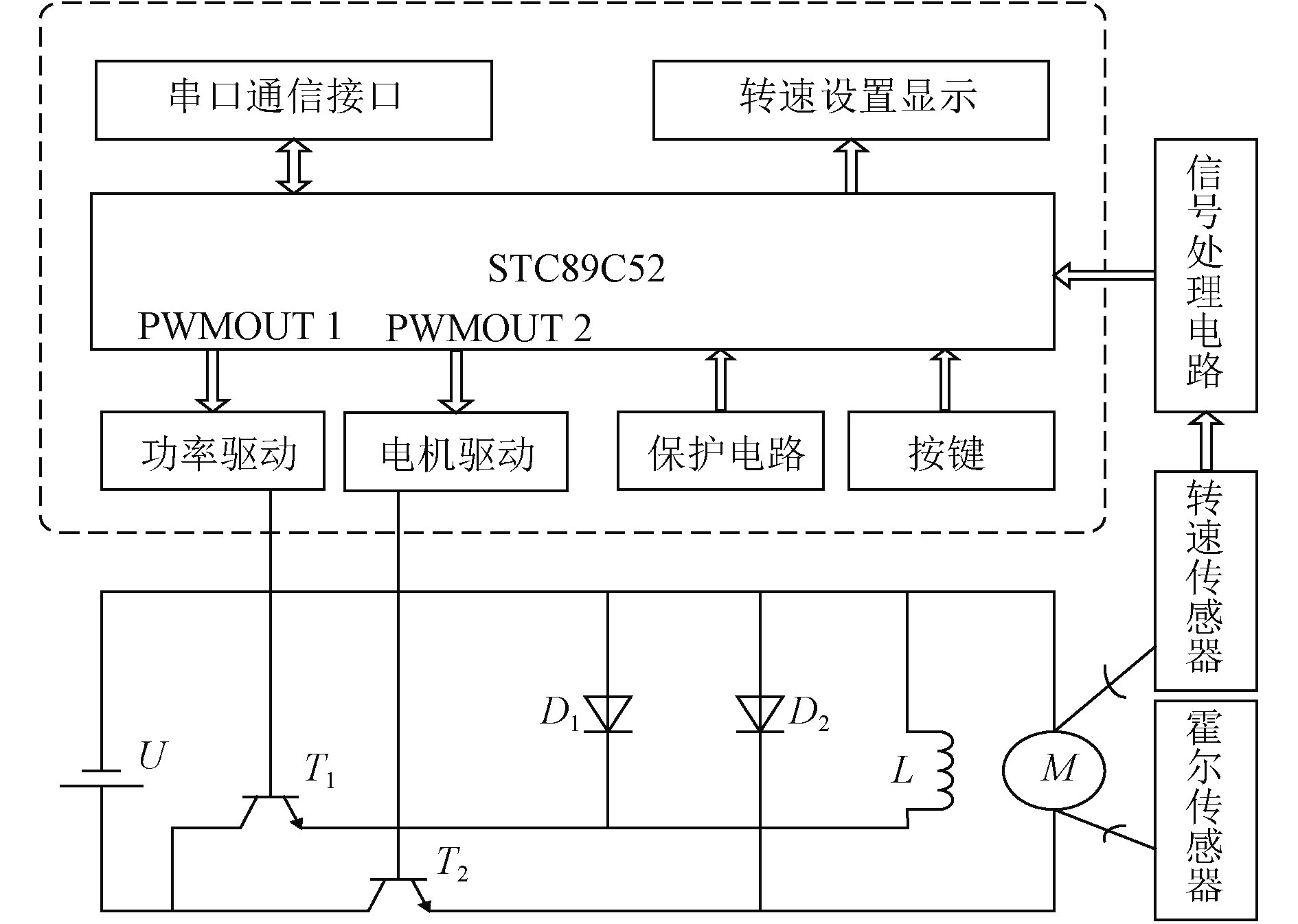图 1 系统框图 Fig. 1 System block diagram

2 闭环PID调节器设计

 ${L_a}\frac{{{\rm d}i}}{{{\rm d}t}} = {u_a} - {i_a}{r_a} - {C_e}\varOmega \left( t \right)\text{，}$ (1)

 $J\frac{{{\rm d}\varOmega \left( t \right)}}{{{\rm d}t}} = T - {T_L}{\text{，}}$ (2)

 $H(s) = \frac{{\varOmega (s)}}{{{U_a}(s)}} = \frac{{{1 / {{C_e}}}}}{{{T_m}{T_a}{s^2} + {T_m}s + 1}}\text{。}$ (3)表 1 模型参数计算公式 Tab.1 Calculation formulas of model parameters表 2 直流电机样机铭牌数据 Tab.2 Nameplate data of DC motor prototype

 $H(s) \!\!=\!\! \frac{{\Omega (s)}}{{{U_a}(s)}} \!\!=\!\! \frac{{{1 / {{C_e}}}}}{{{T_m}{T_a}{s^2} \!+\! {T_m}s \!+\! 1}} \!=\! \frac{{1.565}}{{0.0014{{\rm{s}}^2} + 0.031s + 1}}\text{。}$ (4)

 $u(k) = {K_p}e(k) + {K_i}\sum\limits_{i = 0}^k {e(k) + {K_d}} \left[ {e(k) - e(k - 1)} \right]\text{。}$ (5)

 $\begin{array}{l} \Delta u(k) = u(k) - u(k - 1) =\\ {K_p}\Delta e(k) + {K_i}e(k) + {K_d}[\Delta e(k) - \Delta e(k - 1)] \text{。}\\ \end{array}$ (6)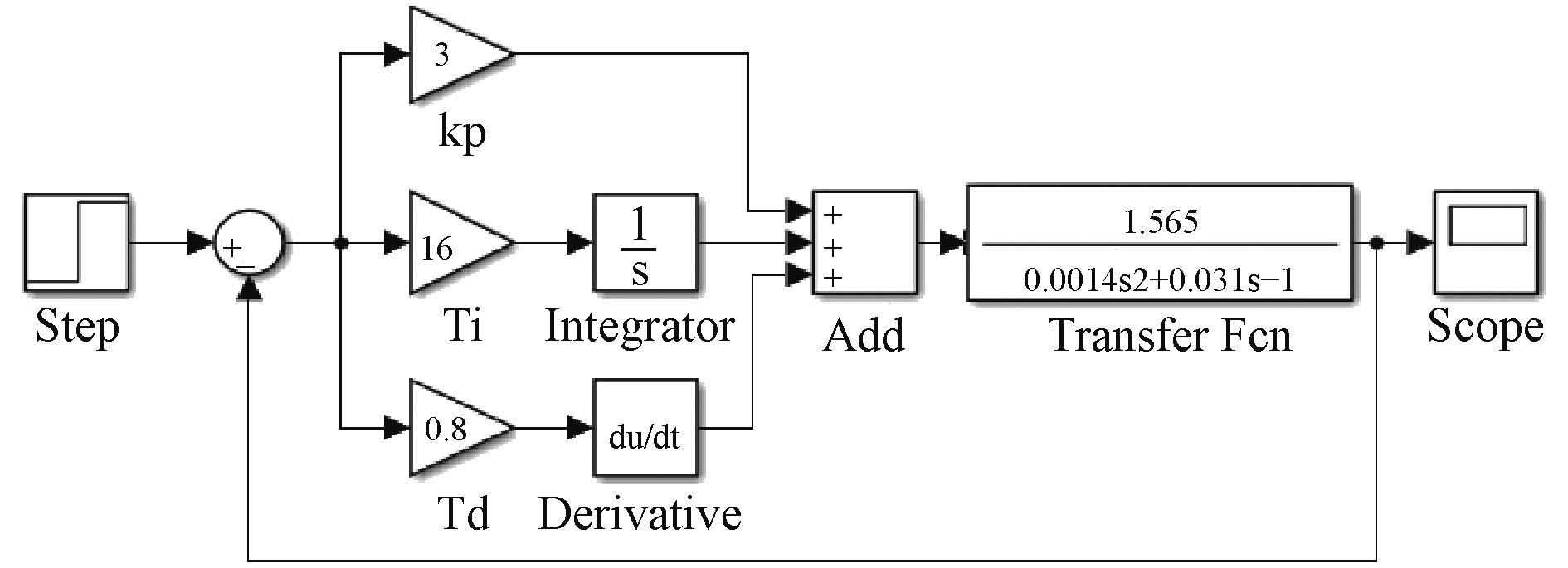图 2 PID参数整定Simulink 模型 Fig. 2 Simulink model of PID parameters tuning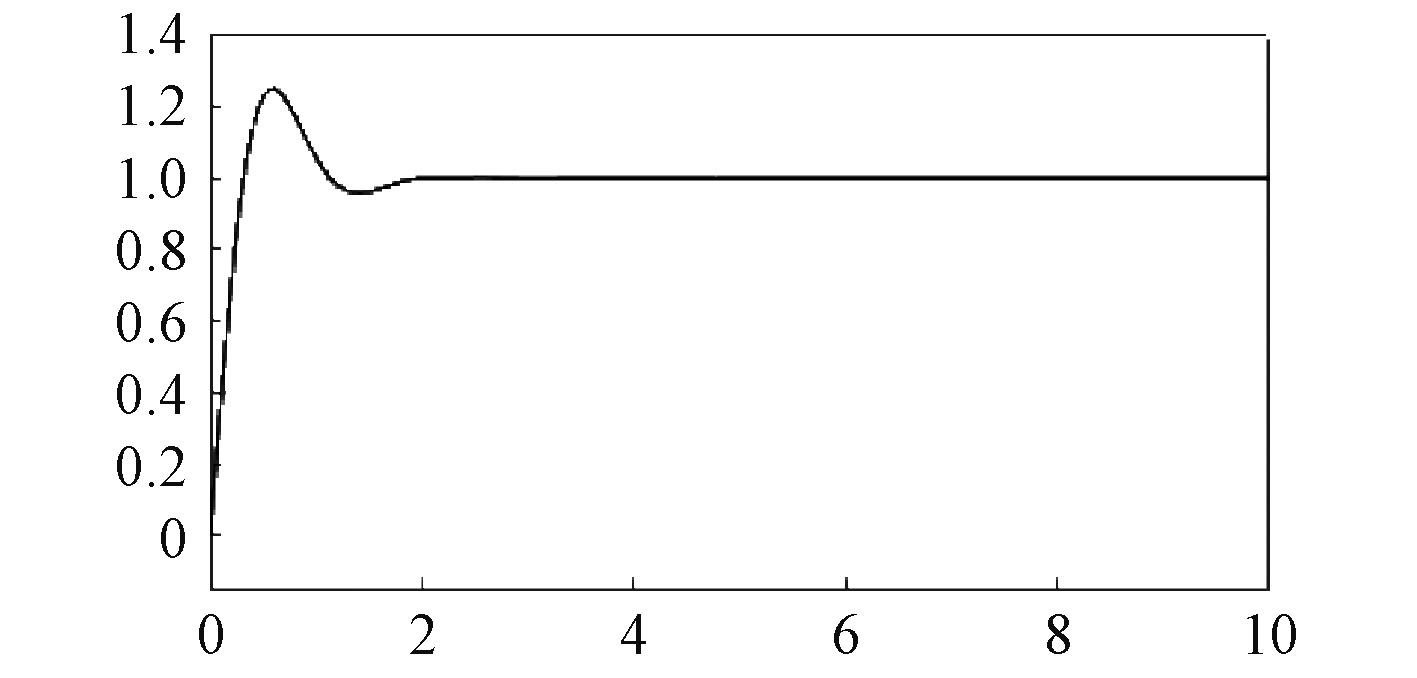图 3 输出响应曲线 Fig. 3 Output response curve
3 电机转速信号处理与系统保护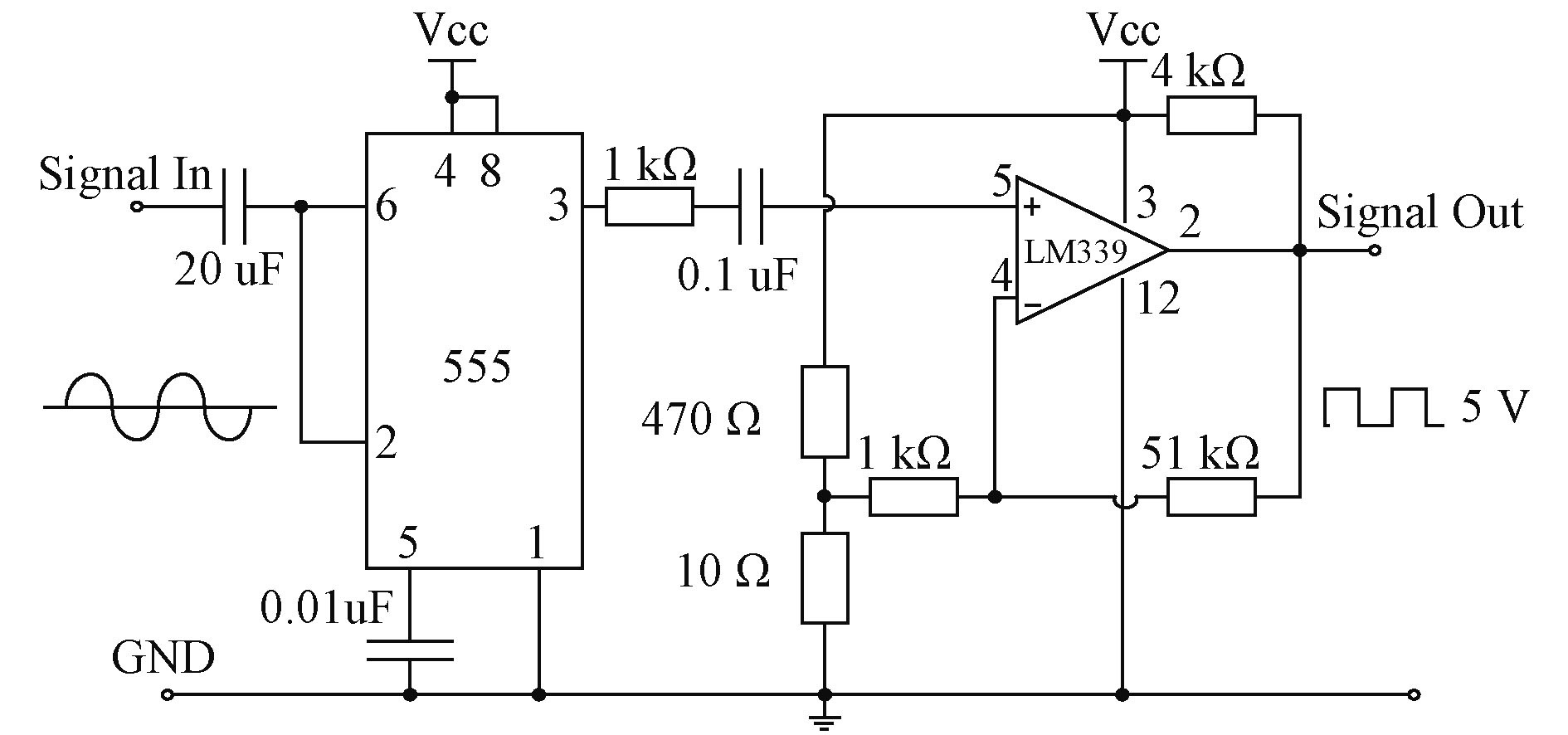图 4 转速信号采集和优化电路 Fig. 4 Speed signal acquisition and optimization circuit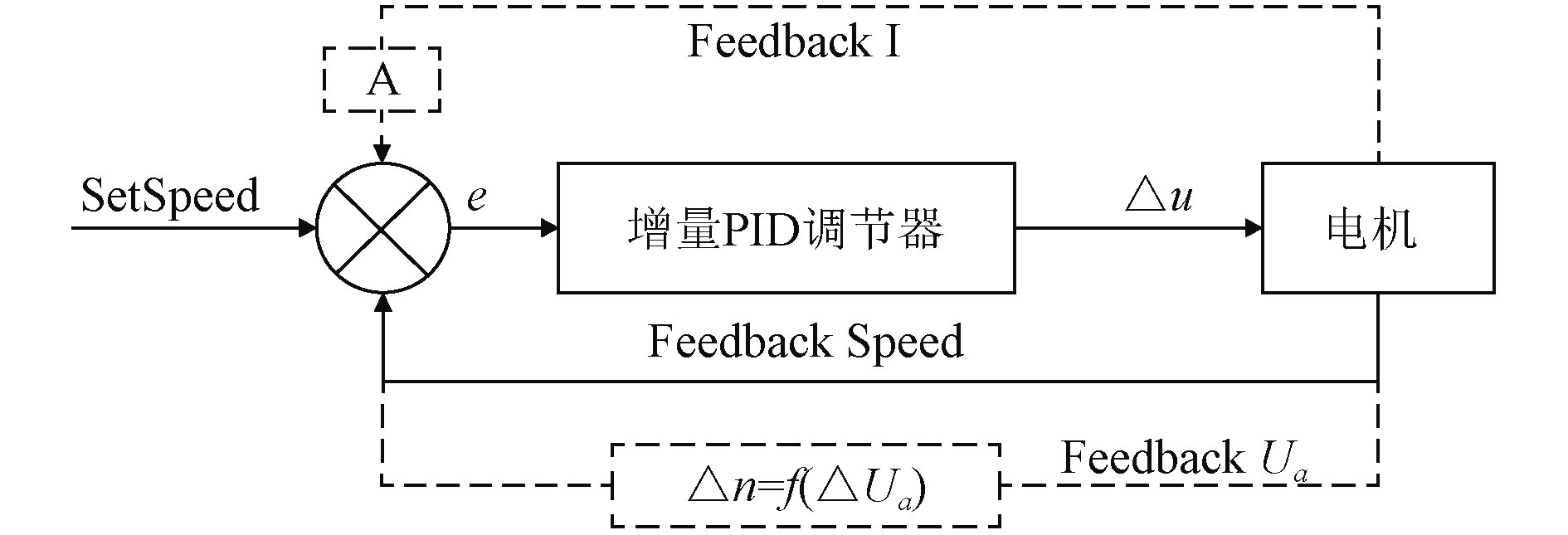图 5 系统控制原理框图 Fig. 5 System control block diagram

4 系统软件设计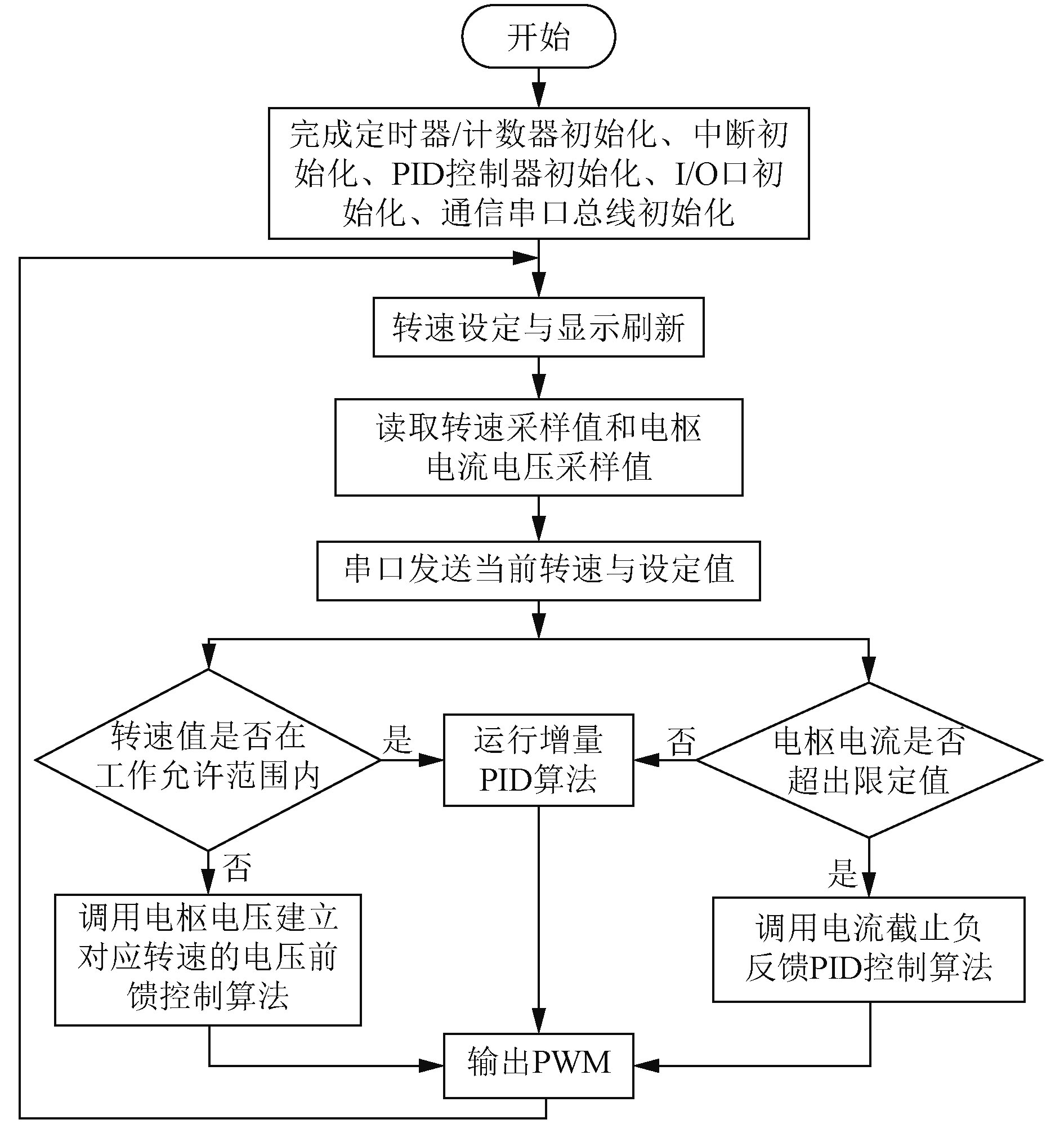图 6 主程序流程图 Fig. 6 Main program flow chart
5 试验分析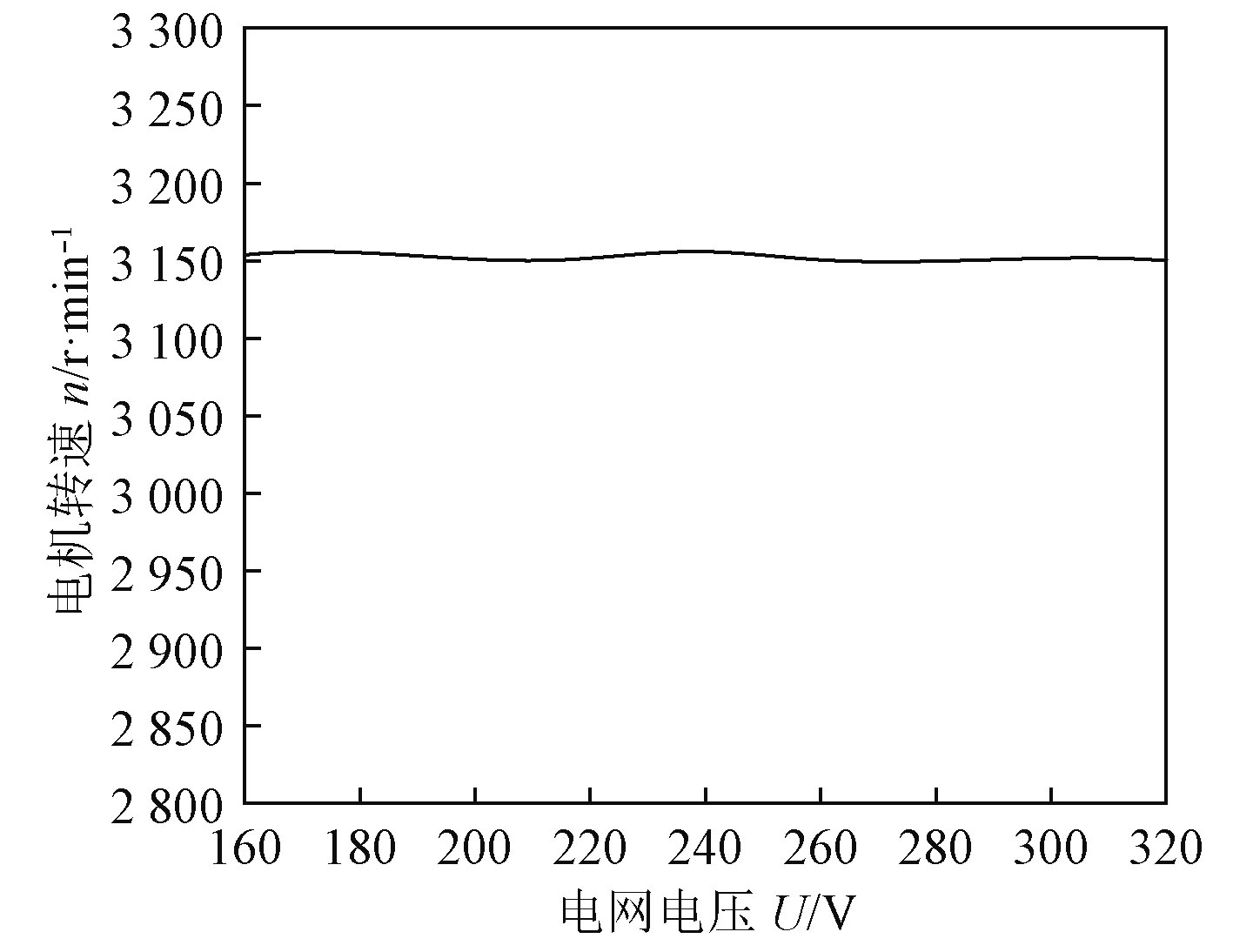图 7 n-U曲线 Fig. 7 n-U curve
6 结　语

  刘金琨. 先进PID控制MATLAB仿真[M]. 北京: 电子工业出版社, 2016.  刘颖. 基于单片机体系的开关磁阻电动机调速系统硬件电路的设计[J]. 煤矿机械, 2013, 11(34): 258-259.  康凯. 基于MATLAB的数字PID直流电机调速系统的实现[J]. 电脑知识与技术, 2010, 6(22): 6372-6373. DOI:10.3969/j.issn.1009-3044.2010.22.099  杨森, 杨杰, 张均东, 等. 基于辩论域模糊PID的船舶柴油发电机调速系统[J]. 中国航海, 2016(1): 16-21.  Swapnil KHUBALKAR, Amit CHOPADE, Anjali JUNGHARE, et al. Design and Realization of Stand-Alone Digital Fractional Order PID Controller for Buck Converter Fed DC Motor[J]. Springer journal, 2016, V35(6): 2189-2211.